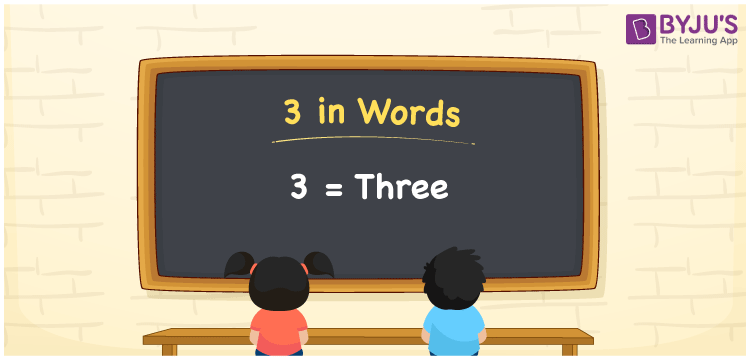# 3 in words

5 in words can be written as Three. The concept of counting is described in the simplest way possible in this article 3 in words, taking in mind the students’ understanding skills. If you spend Rs. 3 for a pencil, you can say, “I purchased a pencil for three rupees.” The conversion of 3 to words is briefly covered here. We can write numbers in words with the help of English Alphabets. Therefore, you can read 3 as “Three” in English.

 3 in words Three Three in Numbers 3

## 3 in English Words## How to Write 3 in Words?

This page discusses the concept of writing 3 in words. In the number 3, one digit is present. The table can be used to understand the place value chart for the one digit of the number 3.

 Ones 3

The number 3 using expanded form can be written as:

3 × One

= 3 × 1

= 3

= Three

Therefore, 3 in words is written as Three.

3 is a natural number that precedes 4 and succeeds 2.

3 in words – Three

Is 3 an odd number? – Yes

Is 3 an even number? – No

Is 3 a perfect square number? – No

Is 3 a perfect cube number? – No

Is 3 a prime number? – Yes

Is 3 a composite number? – No

## Frequently Asked Questions on 3 in Words

Q1

### How can the number 3 be written in words?

3 can be written in words as “Three”.
Q2

### Is 3 an odd number?

Yes, 3 is an odd number as it is not divisible by 2.
Q3

### How can we write Three in numbers?

Three can be written in numbers as 3.